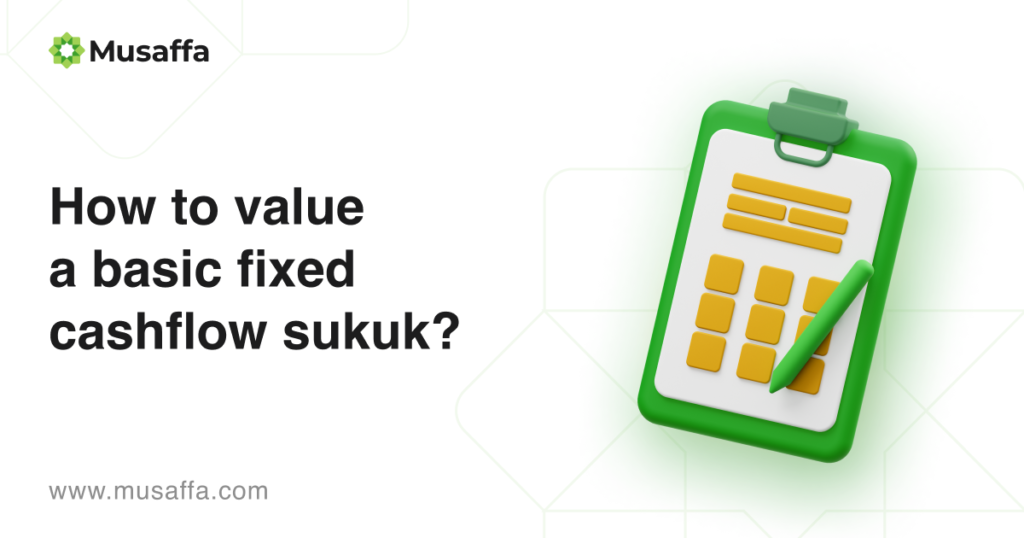# How to Value a Basic Fixed Cash flow Sukuk?What is a basic fixed cash flow Sukuk and how to value it? Sukuk is an Arabic term as a plural form of sakk which means investment or trustee certificate. In the modern-day, Sukuk represents an alternative of bond that pays fixed income to the holders. Given the similarity at a glance, Sukuk is essentially different from the bond in its structure. Only sharia-compliant project is financed by Sukuk. Additionally, it should represent the underlying asset and its value and the existence of a mandatory financing project. These are the main distinctions of Sukuk compared to bonds that likely represent nothing but the issuer’s credibility.

Sukuk carries distributable profit for its holders. Therefore, the value of Sukuk will be the sum of total profit and the par value. Sukuk cannot be issued under the principle of discounted par value. The basis of impermissibility of discounted Sukuk is the unlawfulness of discounting debt. However, Malaysia jurisdiction uses the principle of bay’ al-inah to issue discounted Sukuk. It is a contentious contract that the majority of sharia scholars disallow the use of such a principle to originate Sukuk.

##### Sukuk Ijarah as fixed cash flow Sukuk

Since it represents certain ownership of the underlying asset, different type of Sukuk provides distinct cash flow for its holders. Mudharabah or Musyarakah Sukuk serves somehow fluctuate profit in line with the economic condition. On the other hand, Ijarah Sukuk gives its holders a fixed cash flow because the structure of Sukuk is a rental operation. The basic illustration is if 10 people jointly own a house and rent it to the client for 10 months for USD 100, they will receive a fixed monthly payment of USD 10.

##### Simulation of valuing a basic fixed cash flow sukuk

So far, some countries are still issuing retail Sukuk that is reachable for individual investors. Retail Sukuk is a Sukuk that starts its par value as low as USD 1000, or MYR 1000, or Rp1,000,000, etc. To simulate the calculation, we use a retail Sukuk structure of the Indonesian government. Suppose the sukuk product name is Sukuk Ritel (SR015) originated by the Ministry of Finance. It provides fixed profit at 5.10% per annum with semi-annual payment distribution. The tenor of this sukuk is 3 years from November 2021 until November 2024. Assuming yield-to-maturity (YTM) is 6%, an investment of Rp1,000,000 will have value as follow:

##### Formula

[Value of profits = sum of C/(1 +r)t ] + [Value of principal = F/(1 + r)T]

Where:

F (Face Value)                    = Rp1,000,000

Profit rate                            = 5.10% per annum or 2.55% per period (semi-annual)

C (Future cash flow)         = 2.55 % x Rp1,000,000 = Rp25,500 per period

t (number of periods)     = 3 years x 2 periods = 6

T (time to maturity)        = 6 periods r (yield to maturity)         = 6% per annum or 3% semi-annual

##### Calculation

Present Value of Monthly profit = [Rp25,500/(1+0.03)1] + [Rp25,500/(1+0.03)2] + [Rp25,500/(1+0.03)3] + [Rp25,500/(1+0.03)4] + [Rp25,500/(1+0.03)5] + [Rp25,500/(1+0.03)6] = Rp138,138.381

Present value of principal              = Rp1,000,000/(1+0.03)6 = Rp837,484.256

To sum up, the value of ijarah sukuk is Rp138,138.381 + Rp837,484.256 = Rp975,622.638

##### What does this valuation mean for investors or sukuk holders?

Sukuk holders can refer to this valuation to help them figure out what rate of return would make a Sukuk investment worth the cost. In addition, the valuation indicates the proper pricing to trade Sukuk in the secondary market if Sukuk holders want liquidity or seek potential capital gain. Normally, if the national interest rate decrease, the price of Sukuk will increase and vice versa.